# Importing SAS / SPSS data into R when the variables are defined in another file

I’m trying to import this data into R.

I’m trying to import this data into R.

https://www.cdc.gov/healthyyouth/data/yrbs/data.htm

I know I need the survey package, but these files are odd.

Anyone know what to do?

# From ‘R vs Python’ to ‘R and Python’In this article, you'll learn to leverage the best of both ‘Python and R’ in a single project.

In this article, you'll learn to leverage the best of both ‘Python and R’ in a single project.

If you are into Data Science, the two programming languages that immediately come to mind are R and Python. However, instead of considering them as two options, more often than not, we end up comparing the two. R and Python, are excellent tools in their own right but are very often conceived as rivals. If you type `R vs Python` , in your Google search bar, you instantly get a plethora of resources on topics which talk about the supremacy of one over the other.One of the reasons for such an outlook is because people have divided the Data Science field into camps based on the choice of the programming language they use. There is an R camp and a Python camp and history is a testimony to the fact that camps cannot live in harmony. Members of both the camps fervently believe that their choice of language is superior to the other. So, in a way, divergence doesn’t lie with the tools but with the people using those tools.

### Why not use Both?

There are people in the Data Science community who are using both Python and R, but their percentage is small. On the other hand, there are a lot of people who are committed to only one programming language but wished they had access to some of the capabilities of their adversary. For instance, R users sometimes yearn for the object-oriented capacities that are native to Python and similarly, some Python users long for the wide range of the statistical distributions that are available within R.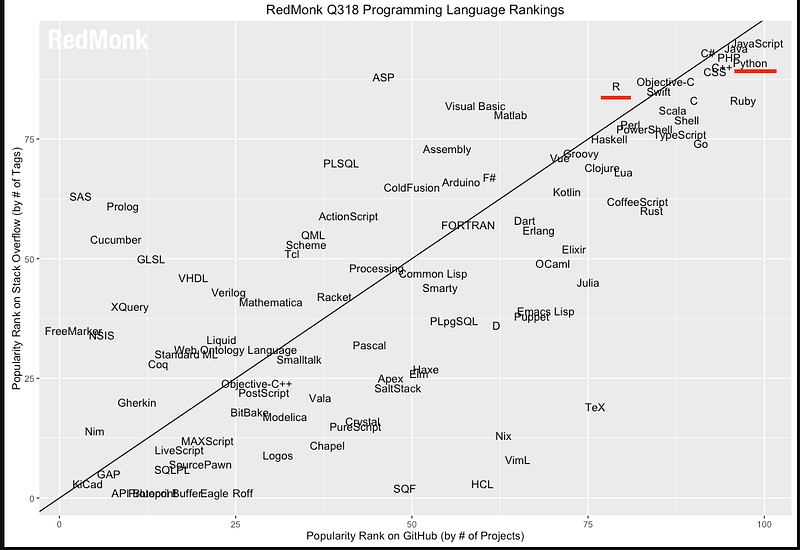The figure above shows the results of the survey conducted by Red Monk in the third quarter of 2018. These results are based on the popularity of the languages on Stack Overflow as well as on Github and clearly show that both R and Python are rated quite high. Therefore, there is no inherent reason as to why we cannot work with both of them on the same project. Our ultimate goal should be to do better analytics and derive better insights and choice of a programming language should not be a hindrance in achieving that.

### Overview of R and Python

Let’s have a look at the various aspects of these languages and what’s good and not so good about them.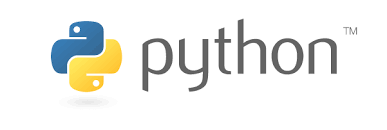#### Python

Since its release in 1991, Python has been extremely popular and is widely used in data processing. Some of the reasons for its wide popularity are:

• Object-oriented language
• General Purpose
• Has a lot of extensions and incredible community support
• Simple and easy to understand and learn
• packages like pandas, numpy and scikit-learn, make Python an excellent choice for machine learning activities.

However, Python doesn’t have specialized packages for statistical computing, unlike R.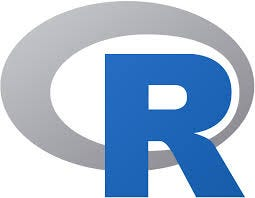#### R

R’s first release came in 1995 and since then it has gone on to become one of the most used tools for data science in the industry.

• Object-oriented language
• General Purpose
• Has a lot of extensions and incredible community support
• Simple and easy to understand and learn
• packages like pandas, numpy and scikit-learn, make Python an excellent choice for machine learning activities.

Performance wise R is not the fastest language and can be a memory glutton sometimes when dealing with large datasets.

### Leveraging the best of Both Worlds

Could we utilize the statistical prowess of R along with the programming capabilities of Python? Well, when we can easily embed SQL code within either R or Python script, why not blend R and Python together?

There are basically two approaches by which we can use both Python and R side by side in a single project.

#### R within Python

• Object-oriented language
• General Purpose
• Has a lot of extensions and incredible community support
• Simple and easy to understand and learn
• packages like pandas, numpy and scikit-learn, make Python an excellent choice for machine learning activities.

PypeR provides a simple way to access R from Python through pipes. PypeR is also included in Python’s Package Index which provides a more convenient way for installation. PypeR is especially useful when there is no need for frequent interactive data transfers between Python and R. By running R through pipe, the Python program gains flexibility in sub-process controls, memory control, and portability across popular operating system platforms, including Windows, GNU Linux and Mac OS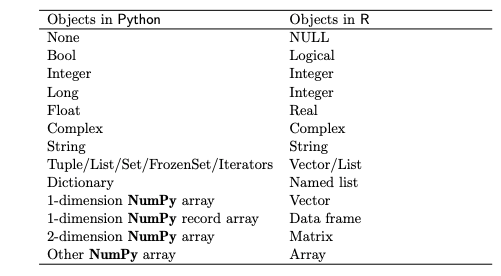• Object-oriented language
• General Purpose
• Has a lot of extensions and incredible community support
• Simple and easy to understand and learn
• packages like pandas, numpy and scikit-learn, make Python an excellent choice for machine learning activities.

pyRserve uses Rserve as an RPC connection gateway. Through such a connection, variables can be set in R from Python, and also R-functions can be called remotely. R objects are exposed as instances of Python-implemented classes, with R functions as bound methods to those objects in a number of cases.

• Object-oriented language
• General Purpose
• Has a lot of extensions and incredible community support
• Simple and easy to understand and learn
• packages like pandas, numpy and scikit-learn, make Python an excellent choice for machine learning activities.

rpy2 runs embedded R in a Python process. It creates a framework that can translate Python objects into R objects, pass them into R functions, and convert R output back into Python objects. rpy2 is used more often since it is one which is being actively developed.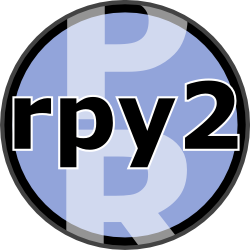One advantage of using R within Python is that we would able to use R’s awesome packages like ggplot2, tidyr, dplyr et al easily in Python. As an example let’s see how we can easily use ggplot2 for mapping in Python.

• Object-oriented language
• General Purpose
• Has a lot of extensions and incredible community support
• Simple and easy to understand and learn
• packages like pandas, numpy and scikit-learn, make Python an excellent choice for machine learning activities.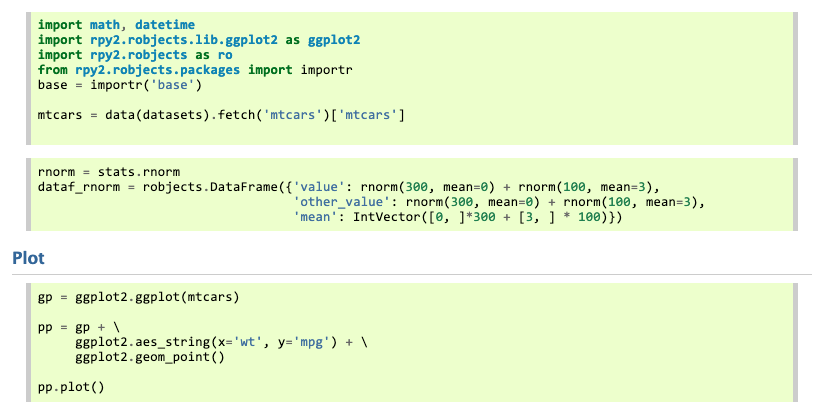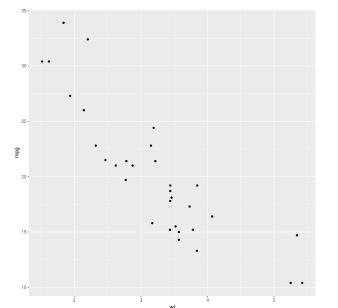• Object-oriented language
• General Purpose
• Has a lot of extensions and incredible community support
• Simple and easy to understand and learn
• packages like pandas, numpy and scikit-learn, make Python an excellent choice for machine learning activities.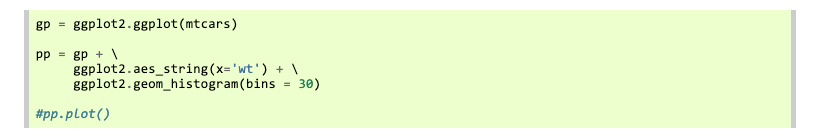[https://rpy2.github.io/doc/latest/html/graphics.html#geometry](https://rpy2.github.io/doc/latest/html/graphics.html#geometry](https://rpy2.github.io/doc/latest/html/graphics.html#geometry) "https://rpy2.github.io/doc/latest/html/graphics.html#geometry](https://rpy2.github.io/doc/latest/html/graphics.html#geometry)")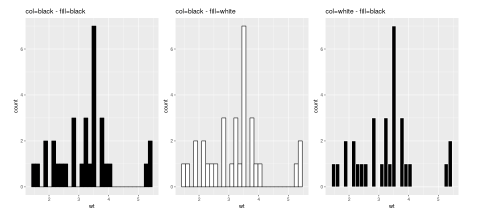#### Resources

You may want to have a look at the following resources for more in-depth review of rpy2:

• Object-oriented language
• General Purpose
• Has a lot of extensions and incredible community support
• Simple and easy to understand and learn
• packages like pandas, numpy and scikit-learn, make Python an excellent choice for machine learning activities.

#### Python with R

We can run R scripts in Python by using one of the alternatives below:

• Object-oriented language
• General Purpose
• Has a lot of extensions and incredible community support
• Simple and easy to understand and learn
• packages like pandas, numpy and scikit-learn, make Python an excellent choice for machine learning activities.

This package implements an interface to Python via Jython. It is intended for other packages to be able to embed python code along with R.

• Object-oriented language
• General Purpose
• Has a lot of extensions and incredible community support
• Simple and easy to understand and learn
• packages like pandas, numpy and scikit-learn, make Python an excellent choice for machine learning activities.

rPython is again a Package Allowing R to Call Python. It makes it possible to run Python code, make function calls, assign and retrieve variables, etc. from R.

• Object-oriented language
• General Purpose
• Has a lot of extensions and incredible community support
• Simple and easy to understand and learn
• packages like pandas, numpy and scikit-learn, make Python an excellent choice for machine learning activities.

SnakeCharmR is a modern overhauled version of rPython. It is a fork from ‘rPython’ which uses ‘jsonlite’ and has a lot of improvements over rPython.

• Object-oriented language
• General Purpose
• Has a lot of extensions and incredible community support
• Simple and easy to understand and learn
• packages like pandas, numpy and scikit-learn, make Python an excellent choice for machine learning activities.

PythonInR makes accessing Python from within R very easy by providing functions to interact with Python from within R.

• Object-oriented language
• General Purpose
• Has a lot of extensions and incredible community support
• Simple and easy to understand and learn
• packages like pandas, numpy and scikit-learn, make Python an excellent choice for machine learning activities.

The reticulate package provides a comprehensive set of tools for interoperability between Python and R. Out of all the above alternatives, this one is the most widely used, more so because it is being aggressively developed by Rstudio. Reticulate embeds a Python session within the R session, enabling seamless, high-performance interoperability. The package enables you to reticulate Python code into R, creating a new breed of a project that weaves together the two languages.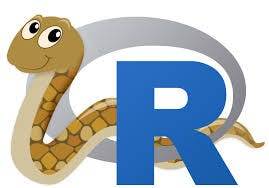The reticulate package provides the following facilities:

• Object-oriented language
• General Purpose
• Has a lot of extensions and incredible community support
• Simple and easy to understand and learn
• packages like pandas, numpy and scikit-learn, make Python an excellent choice for machine learning activities.

#### Resources

Some great resources on using the reticulate package are:

• Object-oriented language
• General Purpose
• Has a lot of extensions and incredible community support
• Simple and easy to understand and learn
• packages like pandas, numpy and scikit-learn, make Python an excellent choice for machine learning activities.

### Conclusion

Both R and Python are quite robust languages and either one of them is actually sufficient to carry on the Data Analysis task. However, there are definitely some high and low points for both of them and if we could utilize the strengths of both, we could end up doing a much better job. Either way, having knowledge of both will make us more flexible thereby increasing our chances of being able to work in different environments.

References:

Interfacing R and Python — Andrew Collier

http://blog.yhat.com/tutorials/rpy2-combing-the-power-of-r-and-python.html

# Data Science Tutorial Using RThis video on "Data Science Tutorial Using R" will give you an in-depth understanding of Data Science and you’ll also learn how Data Science is used in the real world to solve data-driven problems.

Below are the topics covered in this Data Science Course for Beginners:

• Need for Data Science
• Walmart Use case
• What is Data Science?
• Who is a Data Scientist?
• Data Science – Skill set
• Data Science Job roles
• Data Life cycle
• Introduction to Machine Learning
• K- Means Use case
• K- Means Algorithm
• Hands-On
• Data Science certification

# A beginner's guide to R ProgrammingIn this post, you'll learn R Programming for beginner

In this post, you'll learn R Programming for beginner

Introduction

R is a programming language focused on statistical and graphical analysis. It is therefore commonly used in statistical inference, data analysis and Machine Learning. R is currently one of the most requested programming language in the Data Science job market (Figure 1).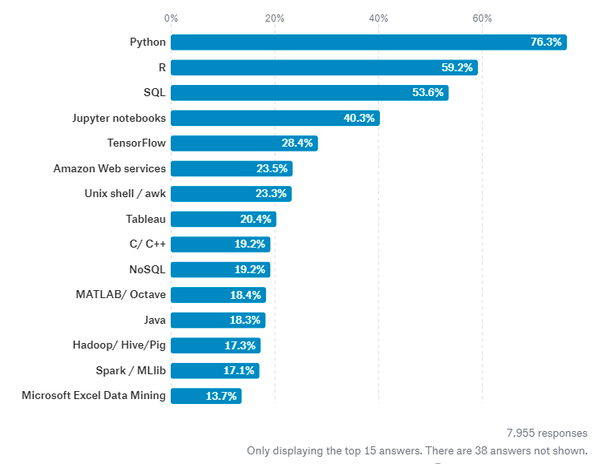Figure 1: Most Requested programming languages for Data Science in 2019 

R is available to be installed from r-project.org and one of R most commonly used integrated development environment (IDE) is certainly RStudio.

There are two main types of packages (libraries) which can be used to add functionalities to R: base packages and distributed packages. Base packages come with the installation of R, distributed packages can instead be downloaded for free using CRAN.

Once installed R, we can then get started doing some data analysis!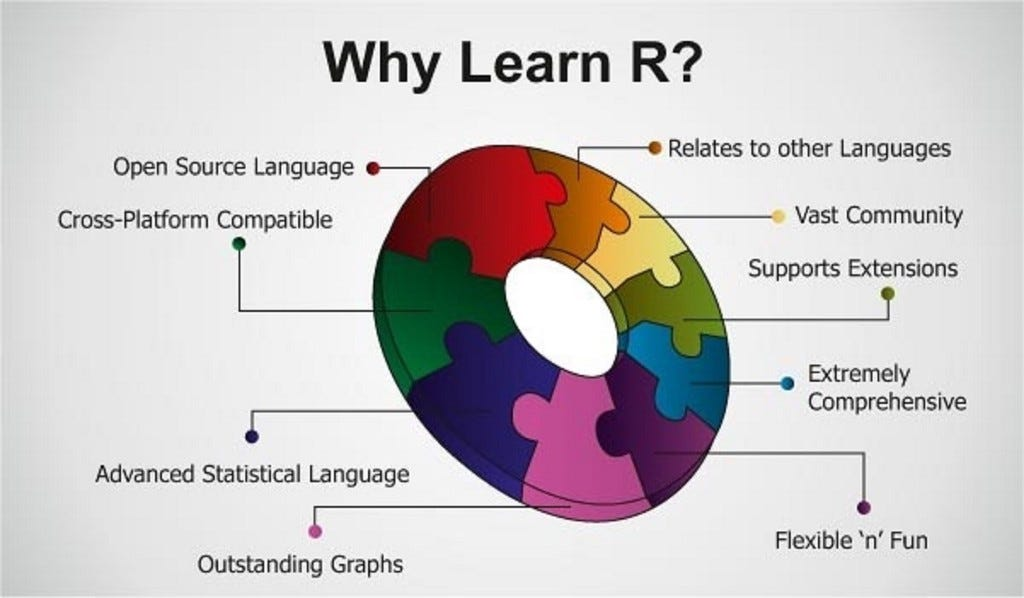Demonstration

In this example, I will walk you through an end to end analysis of the Mobile Price Classification Dataset to predict the price range of Mobile Phones. The code I used for this demonstration is available on both my GitHub and Kaggle account.

Importing Libraries

First of all, we need to import all the necessary libraries.

Packages can be installed in R using the install.packages() command and then loaded using the library() command. In this case, I decided to install first PACMAN (Package Management Tool) and then use it to install and load all the other packages. PACMAN makes loading library easier because it can install and load all the necessary libraries in just one line of code.

``````install.packages("pacman")
library(pacman)
pacman::p_load(pacman, dplyr, ggplot2, rio, gridExtra, scales, ggcorrplot, caret, e1071)

``````

The imported packages are used to add the following functionalities:

• **dplyr: **data processing and analysis.
• **ggplot2: **data visualization.
• **rio: **data import and export.
• **gridExtra: **to make plots graphical objects to which can be freely arranged on a page.
• **scales: **used to scale data in plots.
• **ggcorrplot: to **visualize correlation matrices using ggplot2 in the backend.
• **caret: is used to **train and plot classification and regression models.
• **e1071: **contains functions to perform Machine Learning algorithms such as Support Vector Machines, Naive Bayes, etc…

Data Pre-processing

We can now go on loading our dataset, displaying it’s first 5 columns (Figure 2) and print a summary of the main characteristics of each feature (Figure 3). In R, we can create new objects using the <- operator.

``````# Loading our dataset
df <- import("./mobile_price.csv")

# Getting set of descriptive statistics, depending on the type of variable.
# In case of a Numerical Variable -> Gives Mean, Median, Mode, Range and Quartiles.
# In case of a Factor Variable -> Gives a table with the frequencies.
# In case of Factor + Numerical Variables -> Gives the number of missing values.
# In case of character variables -> Gives the length and the class.
summary(df)

``````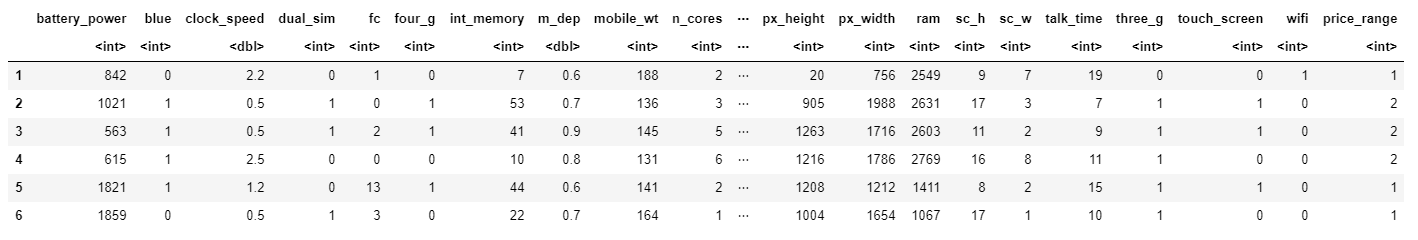The summary function provides us with a brief statistical description of each feature in our dataset. Depending on the nature of the feature in consideration, different statistics will be provided:

• **dplyr: **data processing and analysis.
• **ggplot2: **data visualization.
• **rio: **data import and export.
• **gridExtra: **to make plots graphical objects to which can be freely arranged on a page.
• **scales: **used to scale data in plots.
• **ggcorrplot: to **visualize correlation matrices using ggplot2 in the backend.
• **caret: is used to **train and plot classification and regression models.
• **e1071: **contains functions to perform Machine Learning algorithms such as Support Vector Machines, Naive Bayes, etc…

Factors are a type of data object used in R to categorize and store data (eg. integers or strings) as levels. They can, for example, be used to one hot encode a feature or to create Bar Charts (as we will see later on). Therefore they are especially useful when working with columns with few unique values.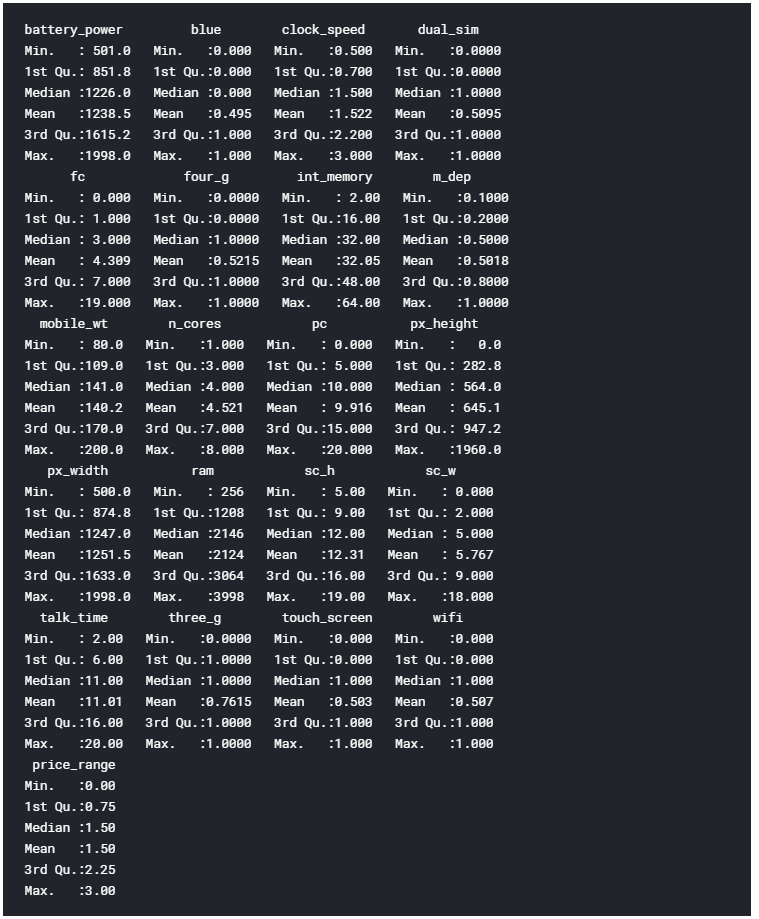Figure 3: Dataset Summary

Finally, we can now check if our Dataset contains any Not A Numbers (NaNs) value using the code shown below.

``````# Checking for Missing values
missing_values <- df %>% summarize_all(funs(sum(is.na(.))/n()))
missing_values <- gather(missing_values, key="feature", value="missing_pct")
missing_values %>%

ggplot(aes(x=reorder(feature,-missing_pct),y=missing_pct)) +

geom_bar(stat="identity",fill="red")+

coord_flip()+theme_bw()

``````

As we can see from Figure 4, no missing numbers have been found.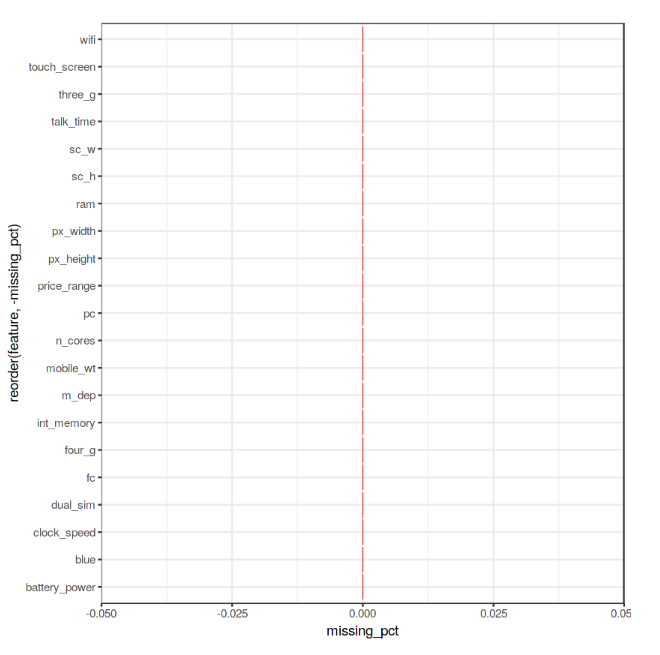Figure 4: Percentage of NaNs in each feature

Data Visualization

We can now start our Data Visualization by plotting a Correlation Matrix of our dataset (Figure 5).

``````corr <- round(cor(df), 8)
ggcorrplot(corr)

``````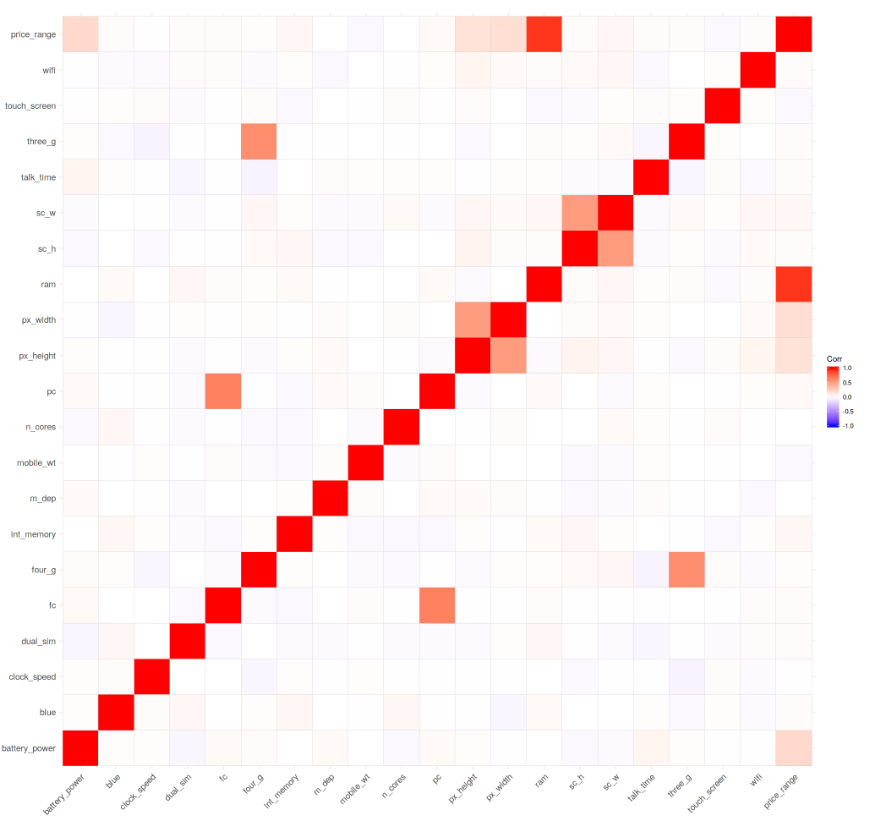Figure 5: Correlation Matrix

Successively, we can start analysing individual features using Bar and Box plots. Before creating these plots, we need though to first convert the considered features from Numeric to Factor (this allow us to bin our data and then plot the binned data).

``````df\$blue <- as.factor(df\$blue)
df\$dual_sim <- as.factor(df\$dual_sim)
df\$four_g <- as.factor(df\$four_g)
df\$price_range <- as.factor(df\$price_range)

``````

We can now create 3 Bar Plots by storing them in there different variables (p1, p2, p3) and then add them to grid.arrange() to create a subplot. In this case, I decided to examine the Bluetooth, Dual Sim and 4G features. As we can see from Figure 6, a slight majority of mobiles considered in this Dataset does not support Bluetooth, is Dual Sim and has 4G support.

``````# Bar Chart Subplots
p1 <-  ggplot(df, aes(x=blue, fill=blue)) +
theme_bw() +
geom_bar() +
ylim(0, 1050) +
labs(title = "Bluetooth") +
scale_x_discrete(labels = c('Not Supported','Supported'))
p2 <- ggplot(df, aes(x=dual_sim, fill=dual_sim)) +
theme_bw() +
geom_bar() +
ylim(0, 1050) +
labs(title = "Dual Sim") +
scale_x_discrete(labels = c('Not Supported','Supported'))
p3 <- ggplot(df, aes(x=four_g, fill=four_g)) +
theme_bw() +
geom_bar() +
ylim(0, 1050) +
labs(title = "4 G") +
scale_x_discrete(labels = c('Not Supported','Supported'))
grid.arrange(p1, p2, p3, nrow = 1)

``````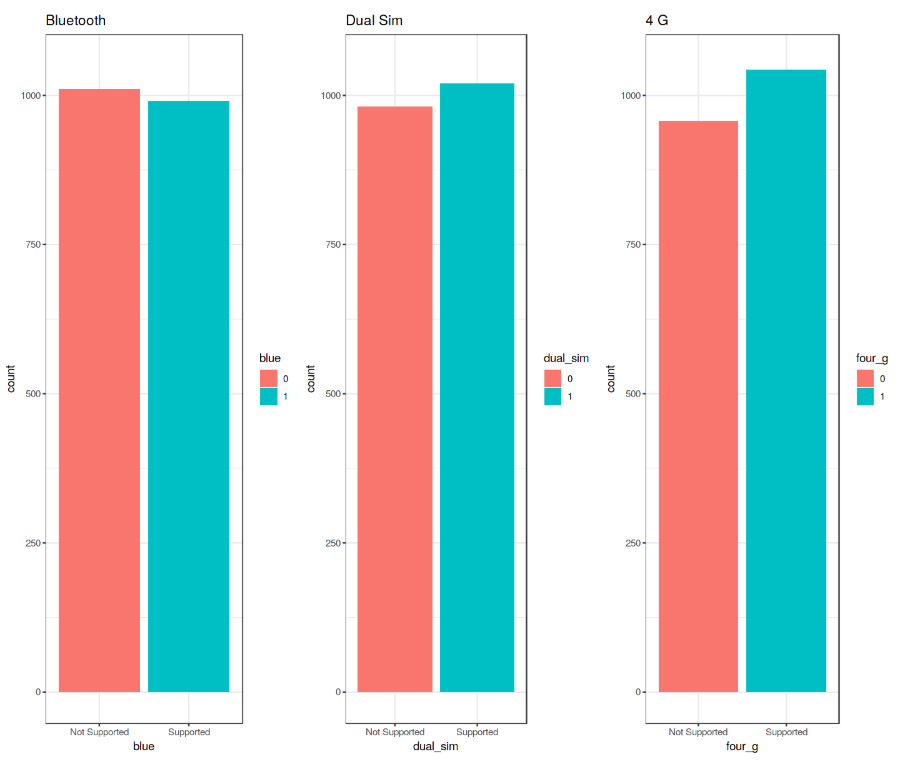Figure 6: Bar Plot Analysis

These plots have been created using R ggplot2 library. When calling the ggplot() function, we create a coordinate system on which we can add layers on top of it .

The first argument we give to the ggplot() function is the dataset we are going to use and the second one is instead an aesthetic function in which we define the variables we want to plot. We can then go on adding other additional arguments such us defining a desired geometric function (eg. barplot, scatter, boxplot, histogram, etc…), adding a plot theme, axis limits, labels, etc…

Taking our analysis a step further, we can now calculate the precise percentages of the difference between the different cases using the prop.table() function. As we can see from the resulting output (Figure 7), 50.5% of the considered mobile devices do not support Bluetooth, 50.9% is Dual Sim and 52.1% has 4G.

``````prop.table(table(df\$blue)) # cell percentages
prop.table(table(df\$dual_sim)) # cell percentages
prop.table(table(df\$four_g)) # cell percentages

``````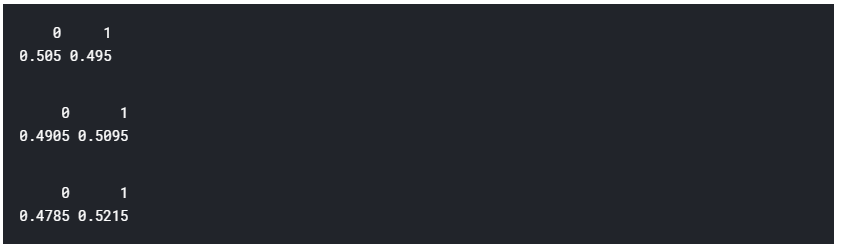Figure 7: Classes Distribution Percentage

We can now go on creating 3 different Box Plots using the same technique used before. In this case, I decided to examine how having more battery power, phone weight and RAM (Random Access Memory) can affect mobiles prices. In this Dataset, we are not given the actual phone prices but a price range indicating how high the price is (four different levels from 0 to 3).

``````# Bar Chart Subplots
p1 <-  ggplot(df, aes(x=price_range, y = battery_power, color=price_range)) +
geom_boxplot(outlier.colour="red", outlier.shape=8,
outlier.size=4) +
labs(title = "Battery Power vs Price Range")
p2 <- ggplot(df, aes(x=price_range, y = mobile_wt, color=price_range)) +
geom_boxplot(outlier.colour="red", outlier.shape=8,
outlier.size=4) +
labs(title = "Phone Weight vs Price Range")
p3 <- ggplot(df, aes(x=price_range, y = ram, color=price_range)) +
geom_boxplot(outlier.colour="red", outlier.shape=8,
outlier.size=4) +
labs(title = "RAM vs Price Range")
grid.arrange(p1, p2, p3, nrow = 1)

``````

The results are summarised in Figure 8. Increasing Battery Power and RAM consistently lead to an increase in Price. Instead, more expensive phones seem to be overall more lightweight. In the RAM vs Price Range plot have interestingly been registred some outliers values in the overall distribution.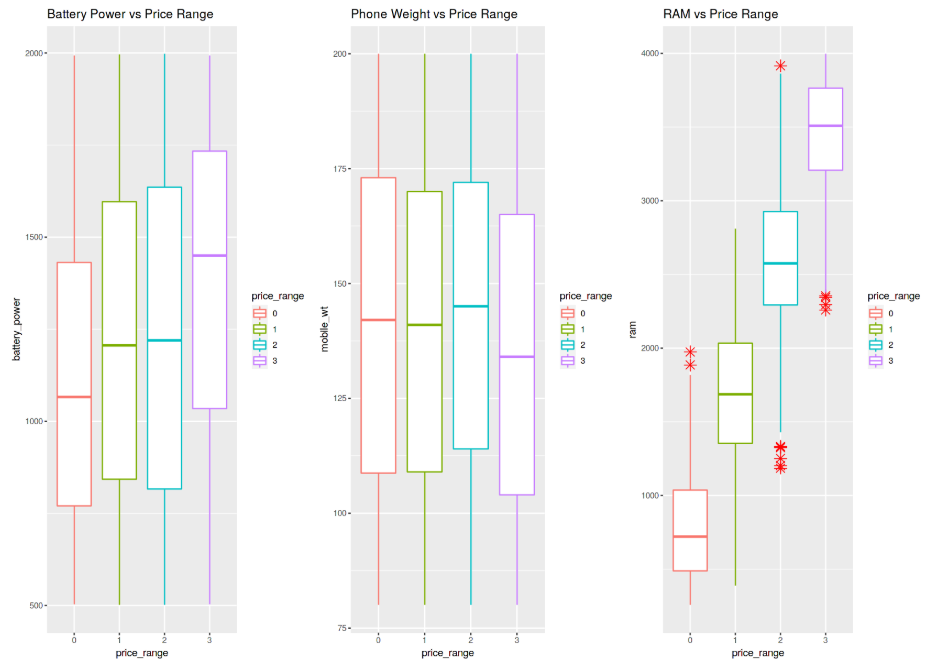Figure 8: Box Plot Analysis

Finally, we are now going to examine the distribution of camera quality in Megapixels for both the Front and Primary cameras (Figure 9). Interestingly, the Front camera distribution seems to follow an exponentially decaying distribution while the Primary camera roughly follows a uniform distribution.

``````data = data.frame(MagaPixels = c(df\$fc, df\$pc),
Camera = rep(c("Front Camera", "Primary Camera"),
c(length(df\$fc), length(df\$pc))))
ggplot(data, aes(MagaPixels, fill = Camera)) +
geom_bar(position = 'identity', alpha = .5)

``````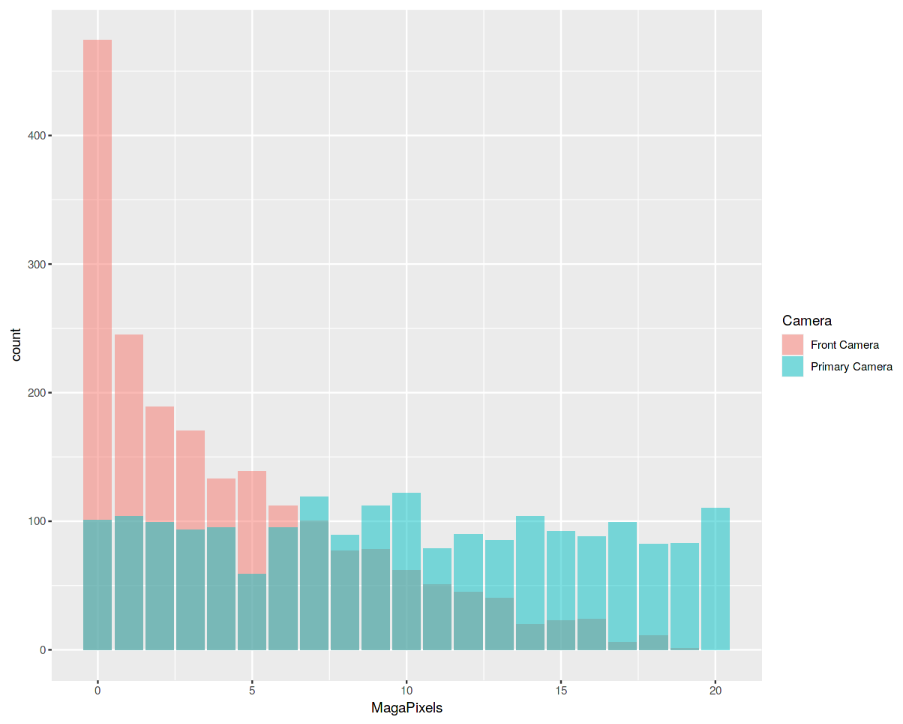Figure 9: Histogram Analysis

Machine Learning

In order to perform our Machine Learning analysis, we need first to convert our Factor variables in Numeric form and then divide our dataset into train and test sets (75:25 ratios). Lastly, we divide the train and test sets into features and labels (price_range).

``````df\$blue <- as.numeric(df\$blue)
df\$dual_sim <- as.numeric(df\$dual_sim)
df\$four_g <- as.numeric(df\$four_g)
df\$price_range <- as.numeric(df\$price_range)

## 75% of the sample size
smp_size <- floor(0.75 * nrow(df))

# set the seed to make our partition reproducible
set.seed(123)
train_ind <- sample(seq_len(nrow(df)), size = smp_size)

train <- df[train_ind, ]
test <- df[-train_ind, ]

x_train <- subset(train, select = -price_range)
y_train <- train\$price_range
x_test <- subset(test, select = -price_range)
y_test <- test\$price_range

``````

It’s now time to train our Machine Learning model. In this example, I decided to use Support Vector Machines (SVM) as our multiclass classifier. Using R summary() we can then inspect the parameters of our trained model (Figure 10).

``````model <- svm(x_train, y_train, type = 'C-classification',
kernel = 'linear')

print(model)
summary(model)

``````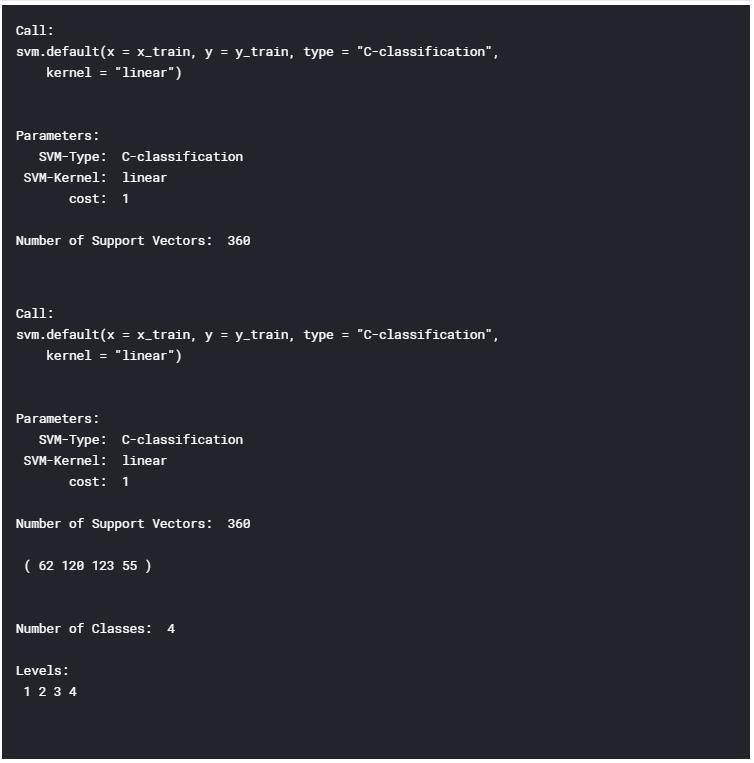Figure 10: Machine Learning Model Summary

Finally, we can now test our model making some predictions on the test set. Using R confusionMatrix() function we can then get a complete report of our model accuracy (Figure 11). In this case, an Accuracy of 96.6% was registered.

``````# testing our model
pred <- predict(model, x_test)

pred <- as.factor(pred)
y_test <- as.factor(y_test)
confusionMatrix(y_test, pred)

``````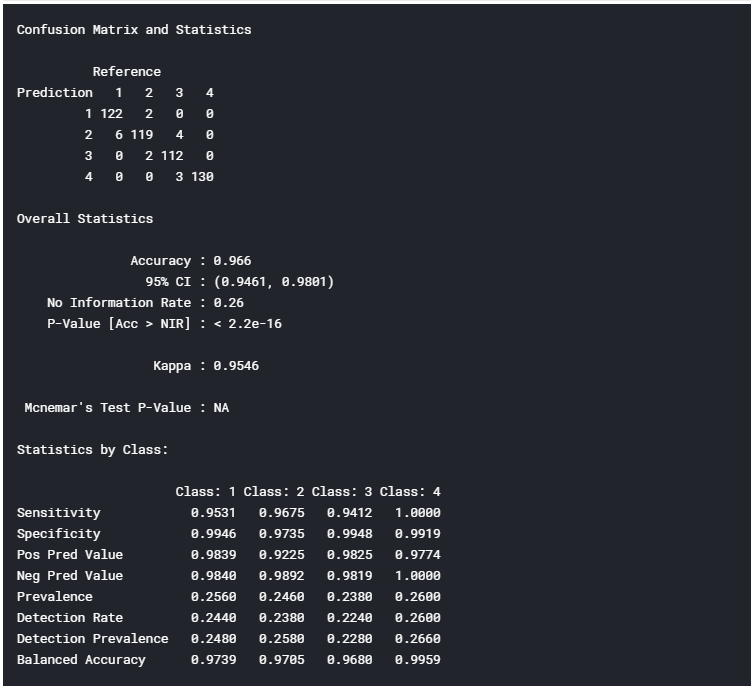Figure 11: Model Accuracy Report

If you liked this post, share it with all of your programming buddies!

# R Programming Tutorial - Learn the Basics of Statistical Computing

Learn the R programming language in this tutorial course. This is a hands-on overview of the statistical programming language R, one of the most important tools in data science.

Thanks for watching

If you liked this post, share it with all of your programming buddies!

# What is R Programming?In this article on What is R programming, I’ll be concentrating on explaining the basic concepts of R.

There are 2.72 million jobs available in the field of data science. R and Python are the two pillars that make playing with data easier. In this article on What is R programming, I’ll be concentrating on explaining the basic concepts of R.

I will cover the following topics in this blog:

• Features of R
• Installing R & RStudio
• R package & libraries
• Variables & Data types
• Operators
• Conditional statements
• Looping statements
• Control Statements
• Functions
• Scope of R Programming

R is an open-source tool used for statistics and analytics. It has become popular in recent years with its applications in the field of Data Analytics, Data Science and Machine Learning among others.

Before we get into features and basics of R Programming, let’s see a scenario where R is used in companies.

Facebook, an online social media-based company aims at improving user engagement, creating and sharing posts. It uses R for exploratory analysis, user engagement analysis, etc. Facebook Data Science group had released a series of blogs that showed an analysis of timeline posts made by users who were Single versus those In a Relationship. The following graph shows the average number of timeline posts exchanged between two people who are about to become a couple.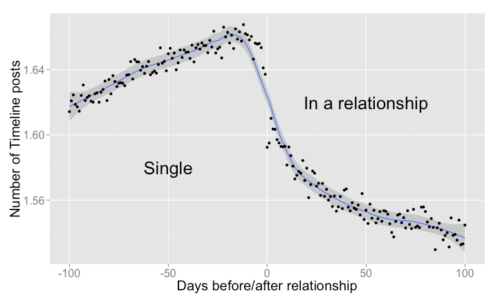The above graph shows the steady change in the number of timeline posts 100 days before and after the relationship. The below graph shows the positive emotions increasing by using tags, words expressing positive emotions.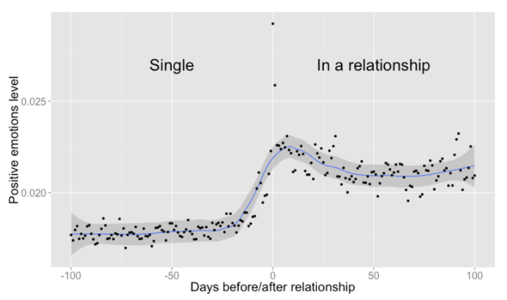Now that we have an idea of what is R, let’s move onto the features of R.

Features of R

Features of R are:

• It is an open-source tool
• R supports Object-oriented as well as Procedural programming.
• It provides an environment for statistical computation and software development.
• Provides extensive packages & libraries
• R has a wonderful community for people to share and learn from experts
• Numerous data sources to connect.

Let’s move ahead to install R and RStudio.

Installing R & RStudio

Go to the R download page and click on the respective **OS, **click on base subfolder. You will find the downloadable link on the top of the page. Run the .exe file and complete the installation by pressing next and install. When you run the R Gui app, the R Console page will be visible at the start.

RStudio is an IDE used for R Programming which is available as open-source and commercial software for Desktop and Server products. Download RStudio Desktop from the RStudio downloads page. On the successful download of the file, run the .exe file and complete the installation. Open the RStudio App and you will see that the entire window is divided into 4 panes as below.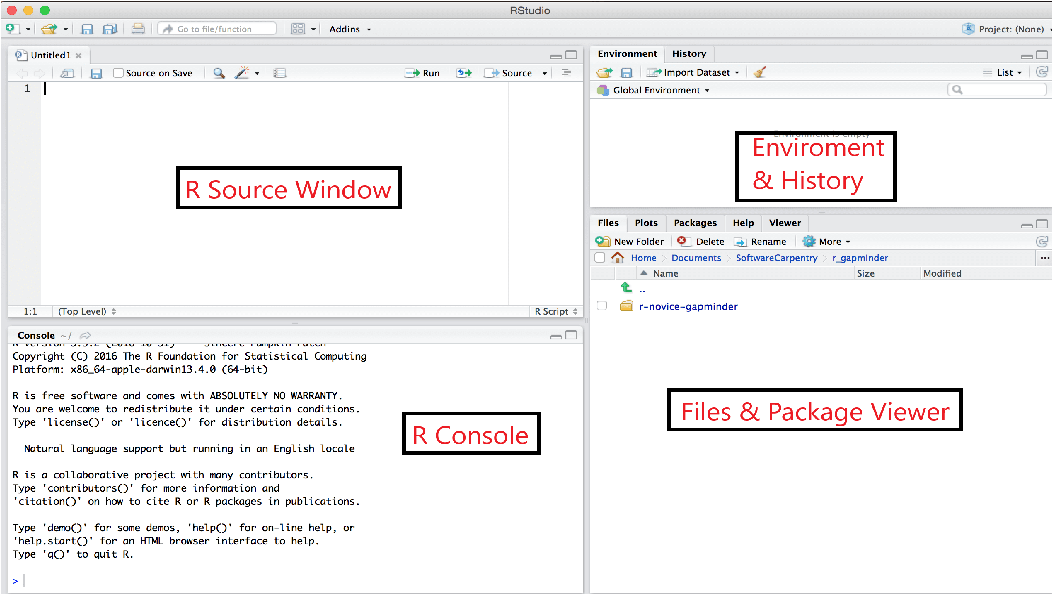### Source window

• We add the source code here and run the whole code by clicking on the source button. To run selected lines, select lines and click Ctrl + Enter or Run button. Run a single line by clicking on CTRL+ Enter.

### R Console

• R displays error logs, warnings, executed statements with their outputs in this pane.

### Environment and History

• This pane consists of 3 tabs. The Environment tab displays all variables defined and used in the R session. The history tab displays the executed statements in R source and Console. The Connections tab display database and external connection-related information.

### Files & Package Viewer

• This pane consists of 5 tabs. The Files tab displays the files in the current working directory. The Plots tab displays graphs, charts created using R packages. The Packages tab lists down installed packages. It also contains 2 buttons (install and update). The Help tab displays the documentation of any package or function in R. The Viewer tab displays web applications and maps that are created using R.

Note: In case any of the 4 panes are closed or hidden, Go to View -> Panes -> Show All Panes to view all panes.

Let’s move forward to learn what is a package and how to load the packages in RStudio.

R package & Libraries

R packages are a group of functions bundled together. These functions are pre-compiled and used in R scripts by preloading them. As discussed above, we can find the list of packages installed in the packages tab at the bottom right window. Let’s learn how to install packages in RStudio.

To install a package, use the following syntax in R Source or R Console.

`install.packages([package-name])`

By default, RStudio installs the packages from CRAN Repository. We can use the functions by loading the package into memory.

To load the package, use the following syntax.

`library([package-name])`

Try Installing the dplyr package in your system and find out what is it used for.

Variables & Data types

### R Variables

Variable is the name of the memory location where data is stored. In other words, we can access memory data using variables.

In R, we can assign variables using any of the following syntaxes. The below-mentioned example assigns the value Edureka to the variable Company.

• Company = “Edureka”
• company <- “Edureka”
• “Edureka” -> CompanY

Note: R variables are case-sensitive.

Variables can be categorized into Continuous and Categorical. If a variable can take on any value between its minimum value and its maximum value, it is called a Continuous variable. Categorical variables (sometimes called a nominal variable) are those that have a fixed number of values or choices such as “Yes”, “No”, etc.

### Datatypes

R consists of 5 main data types: List, Data frame, Vector, Array and Matrix. There are 2 other types called factor and tibble, which are not primary datatypes but will be discussed below.Let’s discuss all the data types in detail.

• List
• Vector
• Array
• Matrix
• Dataframe
• Tibble
• Factor

#### List

A list holds a list of elements. These elements could include either number, decimal number, character, or Boolean value (True/False). They are mutable, i.e., the elements in a list can be modified using the index. A list can also contain a combination of lists, vector, array, and matrix. Let’s learn various list operations –

• Creating a list
List is created using list( ) function. Use the following syntax to create a list.
`list(val1,val2, . . . )`
Example:
``````mylist_1 = list(1, 3.14, "abc", "x")
mylist_1
``````

Output:

``````[]
 1

[]
 3.14

[]
 "abc"

[]
 "x
``````
• You can create a nested list using the same list( ) function. The only difference is that a nested list can have numbers, characters, lists, and other datatype variables.
``````nested_list = list(1,mylist_1,list(1,5,"a"))
``````

Try adding symbols ( \$ . / & ) into a list. [Hint: Escape characters]

Note : Check the data type of variable using class(variable_name).

#### Display list

• Display or print list elements by calling the print( ) function or simply list name.
Example:
``````names = list("Rahul","Nikita","Sindhu","Ram")
names
``````

Output:

``````[]
 "Rahul"

[]
 "Nikita"

[]
 "Sindhu"

[]
 "Ram"
``````
• Accessing List Elements
We access each element within a list using an index. Let’s see some examples of how to access elements.
Example:
``````#Create a list of names.
names = list("Rahul","Nikita","Sindhu","Ram")
#Access first element.
names
``````

Output:

``````[]
 "Rahul"
``````
• Subsetting is the process of accessing several elements. The subset function is used to return subsets of a vector, matrix, or data frame which meets a particular condition. R has powerful indexing features for accessing object elements. These features can be used to select and exclude variables and observations.
The index of an R variable starts from 1 to the length of the list.
Example:
``````#uisng :
names[2:3]
#using vector method.
names[c(2,3)]
``````

Output:

``````[]
 "Nikita"

[]
 "Sindhu"
``````

#### Update list

• Existing elements in a list can be updated by using the element index. Update list elements by assigning a new value to an existing element.
Example:
``````#Update 3rd name in names from Sindhu to Shreya.
names = "Shreya"
names
``````

Output:

``````[]
 "Rahul"

[]
 "Nikita"

[]
 "Shreya"

[]
 "Ram"
``````

• As discussed before, lists are mutable, i.e. list elements can be added as well as be updated. Add a new element into a list using list function or using the length function.
Example:
``````names = "Seetha"
names
``````

Output:

``````[]
 "Rahul"

[]
 "Nikita"

[]
 "Sindhu"

[]
 "Ram"

[]
NULL

[]
 "Seetha"
``````
• Did you see something different from the previous output? That brings us to a question What is NULL?
• NULL represent an element with zero length. Use length function to find the last index and add the element to the list.
``````names[length(names)+1] = "Edureka"
names
``````
• Output:
``````[]
 "Rahul"

[]
 "Nikita"

[]
 "Bindhu"

[]
 "Ram"

[]
 "Edureka"
``````

Try to add NULL into a list at any desired position

• Delete elements
• List elements can be deleted by assigning the element to NULL.
Example:
``````#Delete list elements
names = NULL
names
``````
• Output:
``````[]
 "Rahul"

[]
 "Nikita"

[]
 "Sindhu"
``````

Most of you would have noticed [[ ]] and [ ] in list outputs. Find what is the difference between [[ ]] and [ ].

#### Vector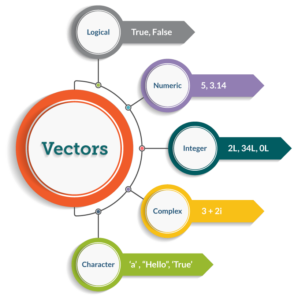A vector is like a list but stores similar types of data, i.e. Numeric, characters or strings, etc. It converts all the elements into a single type depending on the elements in the vector. We can categorize a vector into the below types as shown in the image.

• Numeric Vector (1,808,6527,742,268)
• Integer Vector ( positive and negative real numbers )
• Character vector (“a”, “efjvfVF”, “fbyvkdsb sbv”, “ffWVWVVRV”)
• Logical vector (True/False)
• Complex vector (complex numbers of a+bi form)

Let’s learn vector operations.

### Vector Operations

• Create a vector
• Create a vector using c( ) function. Use the following syntax to create a vector.
`c(val1, val2, ....)`
``````Roll_no = c(1,2,3,4,5)
Roll_no
``````

Output:

`````` 1 2 3 4 5
``````

Note: R has built-in constants. Ex: letters[1:3] = {“a” “b” “c”}, LETTERS[1:3] = {“A” “B” “C”}

The rest operations are the same as a list which brings us to the question: What is the difference between a list and a vector?

#### Difference between list and a vector

• A list holds different data such as Numeric, Character, logical, etc. Vector stores elements of the same type or converts implicitly.
• Lists are **recursive, **whereas vector is not.
• The vector is one-dimensional, whereas the list is a multidimensional object.

#### Array

Array store data in more than two dimensions. It takes vectors as input and uses the values in the dim parameter to create an array.

The basic syntax for creating an array in R is −

`array(data, dim, dimnames)`

Where,

• `data` input vector which becomes the data elements of the array
• `dim` the dimension of the array, where you pass the number of rows, column and the number of matrices to be created by mentioned dimensions
• `dimname` are the names assigned to the rows and columns

Example:

``````v1 = c(9,1,3)
v2 = c(1,7,9,6,4,5)
#Take these vectors as input to the array.
result = array(c(v1,v2),dim = c(3,3,2))
result
``````

Output:

``````, , 1
[,1] [,2] [,3]
[1,]    9   1   6
[2,]    1   7   4
[3,]    3   9   5
, , 2
[,1] [,2] [,3]
[1,]    9   1   6
[2,]    1   7   4
[3,]    3   9   5
``````

What is the difference between NA and NULL?

Note: Check out the number of rows and columns of R object using nrow(var) and ncol(var).

#### Matrix

A matrix is a collection of data elements arranged in a two-dimensional rectangular layout.

The syntax to create a matrix is –

`matrix(data, nrow, ncol, byrow, dimnames)`

Where:

• `data` is the input vector,
• `nrow` the number of rows to be created
• `ncol` is the number of columns to be created
• `byrow` is a logical clue. If TRUE, then the input vector elements are arranged by row
• `dimname` names assigned to the rows and columns

Example:

``````A = matrix(c(2, 6, 3, 1, 5, 7),nrow=2,ncol=3,byrow = TRUE)
A
``````

Output:

``````     [,1] [,2] [,3]
[1,]   2    6    3
[2,]   1    5    7
``````

#### Data Frame

A Data Frame is a table-like structure that contains rows and columns. A data frame can be created by combining vectors.

The basic syntax for creating a data frame using is

`data.frame(vect1, vect2, ...)`

Example:

``````id = c(1:5)
names = c("Srinath","Sahil","Anitha","Peter","Siraj")
employees = data.frame(Id = id, Name = names)
employees
``````

Output:

``````  Id Name
1 1 Srinath
2 2 Sahil
3 3 Anitha
4 4 Peter
5 5 Siraj
``````

#### Characteristics of a data frame

• The column names should be non-empty
• Each column should contain the same amount of data items
• The data stored in a data frame can be of numeric, factor or character type
• The row names should be unique

Note: Check out description of any variable using str(variable)

### Tibble

A Tibble is a table-like structure similar to a data frame. Create a tibble variable using the following syntax:

`tibble(list1,list2, ... )`

Example:

``````id = c(1:5)
names = c("Srinath","Sahil","Anitha","Peter","Siraj")
employees = tibble(Id = id, Name = names)
employees
``````

Output:

``````# A tibble: 5 x 2
Id Name
<int> <chr>
1    1 Srinath
2    2 Sahil
3    3 Anitha
4    4 Peter
5    5 Siraj
``````

Let’s find out what makes a tibble different from the data frame.

#### Differences between Tibble and Data Frame

• Tibble displays data along with the data type whereas a data frame display data only
• Tibble fetches data from the data source in its original data type. Dataframe fetches data from the data source as factors if data types are not specified
• Tibble is stricter than data frames in slicing. Slicing is a list/vector operation to return a slice in a given R object(vector, data frame)

Note: Check out dimensions of any variable using dim(var).

### *actor

A factor is another data type that is created while reading data from external data sources. While loading CSV or text files, it converts any column with categorical values to factor. Any vector can be converted to factor using below syntax:

Syntax:

``````as.factor(vector)
``````

A factor converts categorical values into a numerical vector with multiple levels.

Example:

``````as.factor(names)
``````

Output:

`````` Rahul Nikita Sindhu Ram
Levels: Nikita Rahul Ram Sindhu
``````

Now we have learned different data types of R. Let’s move ahead and learn about operators in R programming.

Operators

R supports the following operators,

#### Arithmetic Operators• Relational Operators• Logical Operators### Assignment Operators

Assignment operator assigns value or variable to operand.

The assignment operators are =, <-, ->.

Examples:

``````10 -> b
a = 5
c <- a+b
``````

We have covered different operators used in R Programming, now let’s understand various Conditional, Looping and Control statements.

Conditional statements

R comprises 3 conditional statements which are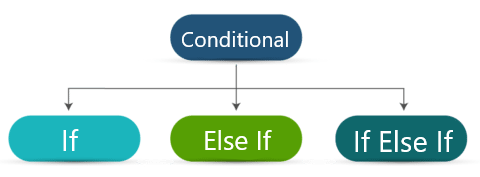Lets us discuss them individually.

### If Statement

The flow of If statement: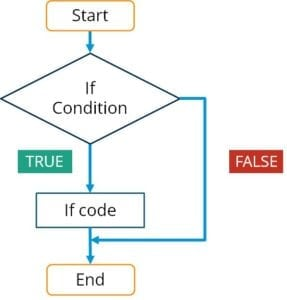As shown in the above picture, if the condition is true, then execute If code else executes the statements that come after if body.

Syntax:

`if(condition) {`

`If code`

`}`

`statements`

Example:

``````Grade = "Good"
print("Good")
}
``````

Output:

`````` "Good"
``````

### Else If Statement

The flow of Else If Statement: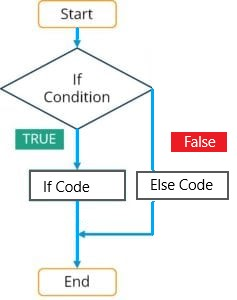As shown in the above picture, if the condition is true, then execute If code else executes Else code and then follow the statements that come after the if-else body.

Syntax:

`if(condition) {`

`If code`

`}`

`else {`

`Else code`

`}`

`Statements`

Example:

``````Grade = "Good"
print("Good")
}
else {
}
``````

Output:

`````` "Good"
``````

### If Else If Statement

The flow of If Else If Statement: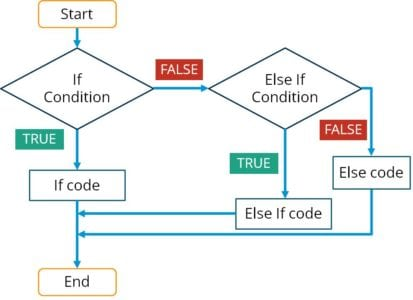As shown in the above picture, if the condition is true, then execute If code else checks the second condition. If the condition is true, execute Else If code otherwise executes **Else code **followed by statements that come after if-else-if body.

Syntax:

`f(condition) {`

`If code`

`}`

`else if (condition){`

`Else if code`

`}else {`

`Else code}`

Example:

``````Grade = "OK"
print("Good")
}
print("Ok")
}
else {
}
``````

Output:

`````` "Ok"
``````

Switch statement

A switch is another conditional statement used in R. If statements are generally preferred over switch statements. The basic syntax of the switch statement is –

Syntax:

`switch (expression, list)`

Example:

``````switch(2,"GM","GA","GN")
``````

Output:

`````` "GA"
``````
Looping statements

Looping statements reduce the work of a user to perform a task multiple times. These statements execute a segment of code repeatedly until the condition is met.

R comprises 3 looping statements which are,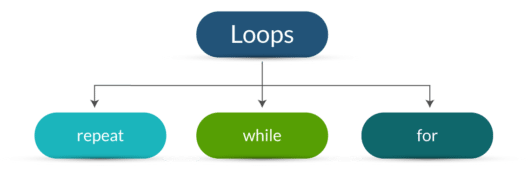Lets us discuss each in detail.

For Loop

For loop is the most common looping statement used for repeating a task. A for loop executes statements for a known number of times. Define a for loop using the following syntax:

Syntax:

`for(var in range){`

`statements`

`}`

Example:

``````for(x in 1:10){
print(x)
}
``````

Output:

`````` 1
 2
 3
 4
 5
 6
 7
 8
 9
 10
``````

While Loop

A while loop repeats a statement or group of statements until the condition is true. It tests the condition before executing the loop body. A while loop is created using the following syntax:

Syntax:

`while(condition) {`

`Statement`

`}`

Example:

``````a = 5
while(a>0) {
a=a-1
print(a)
}
``````

Output:

`````` 4
 3
 2
 1
 0
``````

#### Repeat

Repeat loop is the best example of an exit controlled loop where the code is first executed and then the condition is checked to determine if the control should be inside the loop or exit from it. Create a repeat loop using the following syntax:

Syntax:

`repeat {`

`statements`

`if(condition) {`

`statements`

`}`

`}`

Example:

``````m=5
repeat {
m= m+2
print(m)
if(m>15) {
break
}
}
``````

Output:

`````` 7
 9
 11
 13
 15
 17
``````

Control statements

R has the following control statements,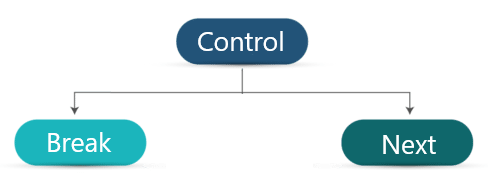Lets us discuss each in detail.

#### Break

A break statement is used to stop or terminate the execution of statements. When the break statement is encountered inside a loop, the loop is immediately terminated and program control resumes at the next statement following the loop. If else and switch statements contain break statements usually to stop the execution. The syntax to use the break statement is –

Syntax:

`break`

Example:

``````m=5
repeat {
m= m+2
print(m)
if(m>15) {
break
}
}
``````

Output:

`````` 7
 9
 11
 13
 15
 17
``````

#### Next

The next statement is used to skip the current iteration of a loop without terminating or ending it. The syntax of the next statement is

Syntax:

`next`

Example:

``````for(i in c(1:6)) {
if (i == "3") {
next
}
print(i)
}
``````

Output:

`````` 1
 2
 4
 5
 6
``````
Functions

A function is a set of statements to perform a specific task. R has in-built functions and also allows the user to create their own functions. A function performs a task and returns a result into a variable or print the output in the console.

R contains two types of functions,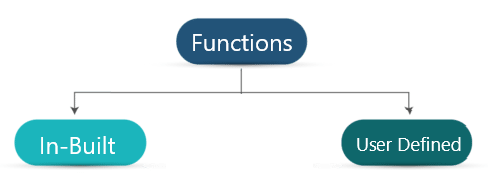#### Built-in Functions

Built-in functions are those pre-defined in R such as mean, sum, median, etc.

#### User-Defined Functions

User-Defined functions are defined as per the requirements. Define a function using the following syntax:

### Function definition

`function_name <- function(arg_1, arg_2, ...) {`

`Function body`

`}`

Store the function definition in a variable and call the function using variable followed by optional parameters inside the parenthesis ( ).

Example

``````factorial <- function(n) {
if(n<= 1) { return(1)
}
else {
return(n * factorial(n-1))
}
}
factorial(3)
``````

Output:

`````` 6
``````
Scope of R programming

In this busy world, everybody learns a new language or technology for the sake of career, fame or salary. Before learning or taking up any course, this question would come to anyone’s mind “What is R Programming and why to learn R over other technologies and tools?”.

R has an excellent growth in various aspects such as Career growth, Job aspect, Business requirements, Cost, Salary, etc. It is open source and has been gaining a lot of audiences lately. It reduces half the burden to buy a licensed product. R is an All in one tool that not only performs analysis but is also used in making reports, dashboards, applications, etc. let’s discuss a few aspects of “why to learn R?’.

### Salary

The need for people with R skills is increasing and so is the salary. Salary of engineers or programmers working with R varies between 3.9LPA to 20LPA. As shown in the image below.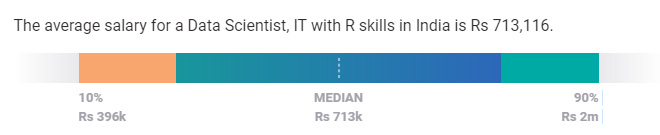Source: Payscale.

### Job roles

The number of jobs available for R Programmers is increasing in recent years. There are different roles available for people with R Programming skills such as:

1. Data Scientist
2. Data Analyst
3. R Programmer/ Developer